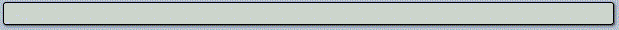## Validating Predictors

Parallax has been producing neural network-based financial predictors since 1990, so it is an integral part of our business to validate these predictors using reliable statistical methods. With each predictor, we need to answer the following set of questions:

1. What price behavior is being predicted?
2. What is the effective duration of the predictor?
3. How statistically significant is the predictor at each time step forward?
4. Is the predictor effective on all time scales?
5. Is the predictor effective on all financial series?

The following figure shows a simple and reliable statistical method to analyze eight years of data for one of our predictors. In order to carry out this analysis, it is necessary to measure the price action during the time period immediately following each signal. We call this the post-predictor or outrun period.

In this case we chose to measure the number of standard deviations (Z) at bar eleven of the outrun period, accumulate them in a histogram, and then statistically compare these to a randomly selected background from the same eight-year period. The Chi-squared test we performed yielded a p-value of 5.4E-8, which means that there is only a 1 in 18 million chance of our results being random. Here is the distribution of buy signals from ExtremeHurst v4 versus the backgound:

####and the median and average percent gain on daily scale equity charts: